# Deep Q­-Learning

## Q-Learning

$$Q\left(s_{t}, a_{t}\right)=\max R_{t+1}$$

$$\pi(s)=\operatorname{argmax}_{a} Q(s, a)$$

$$Q_{i+1}(s, a)=r+\gamma \max _{a^{\prime}} Q_{i}\left(s^{\prime}, a^{\prime}\right)$$

Q-Learning 的核心思想：用 Bellman 方程迭代近似估计 Q-函数。一种最简单的方式实现 Q-函数 的方式就是建立一个行为状态，列为动作的表格。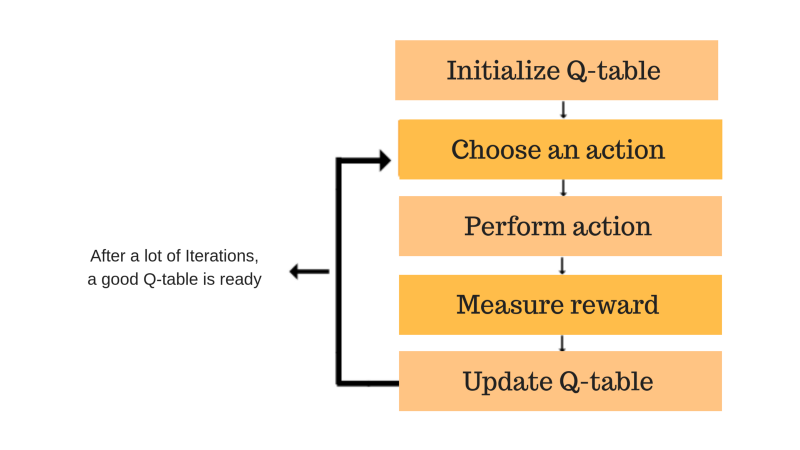Q-learning 伪代码表示如下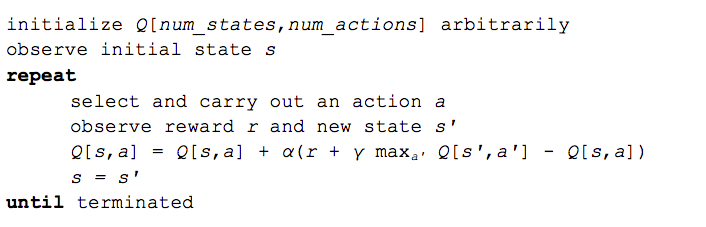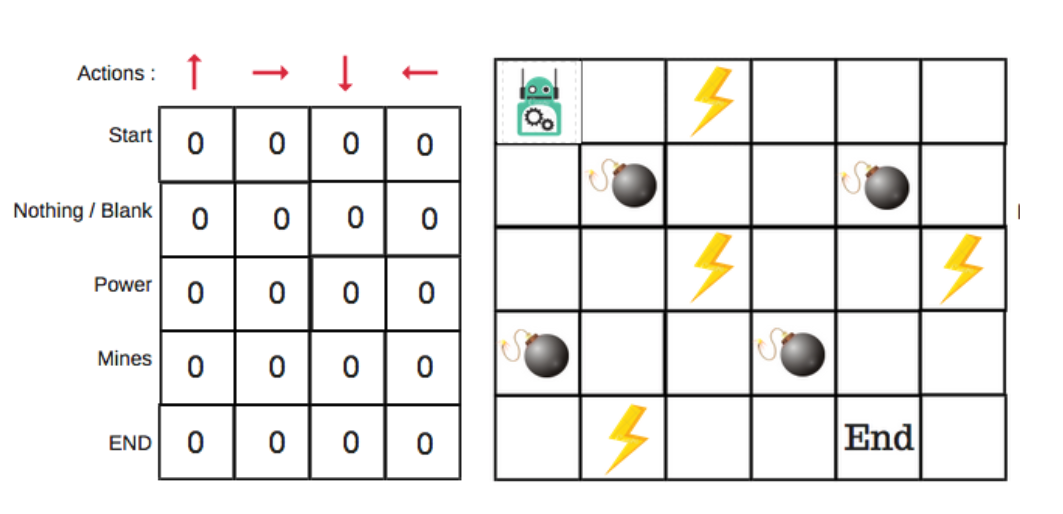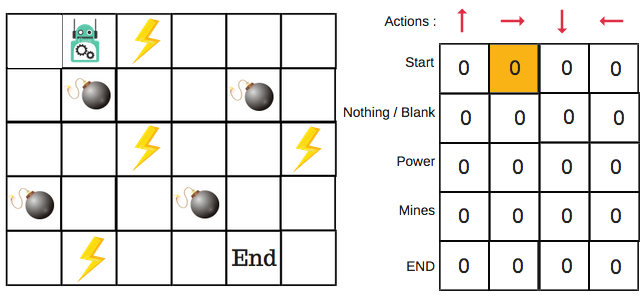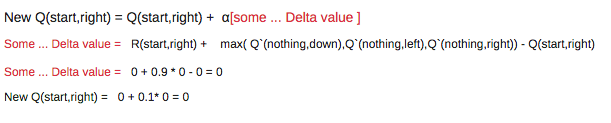## 深度 Q 网络(Deep Q Network)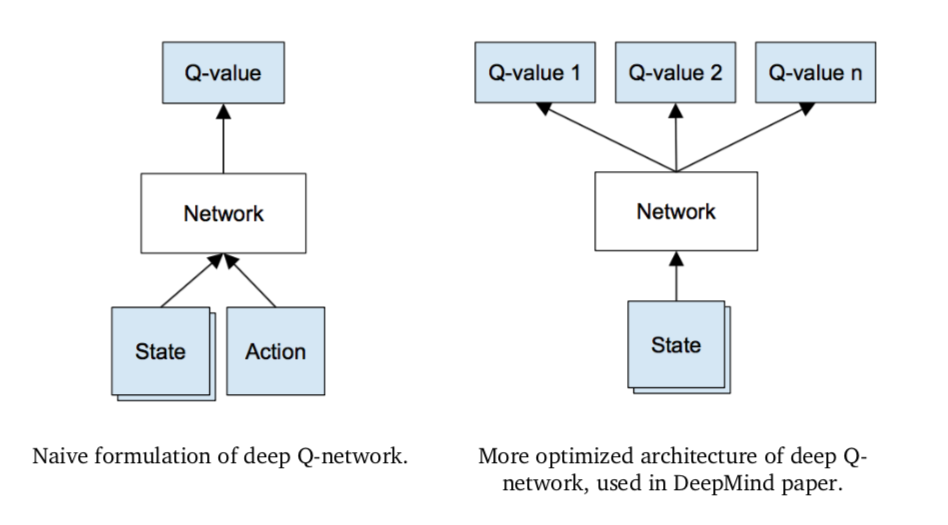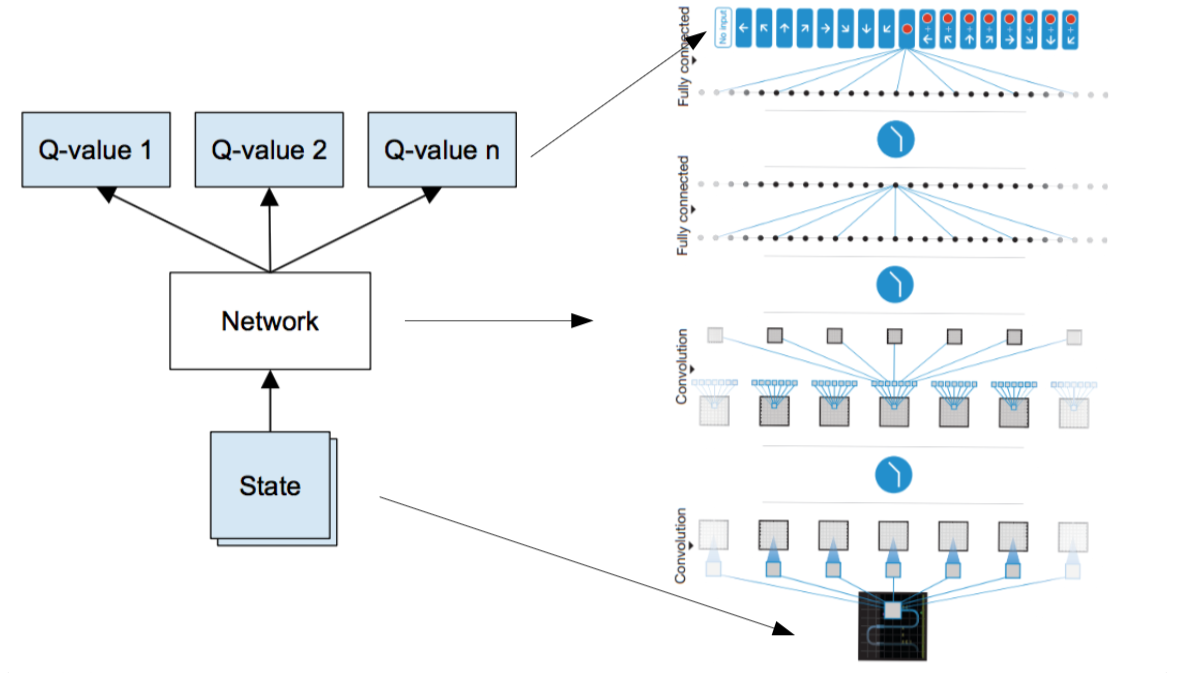DeepMind 在文章中使用的网络模型如下。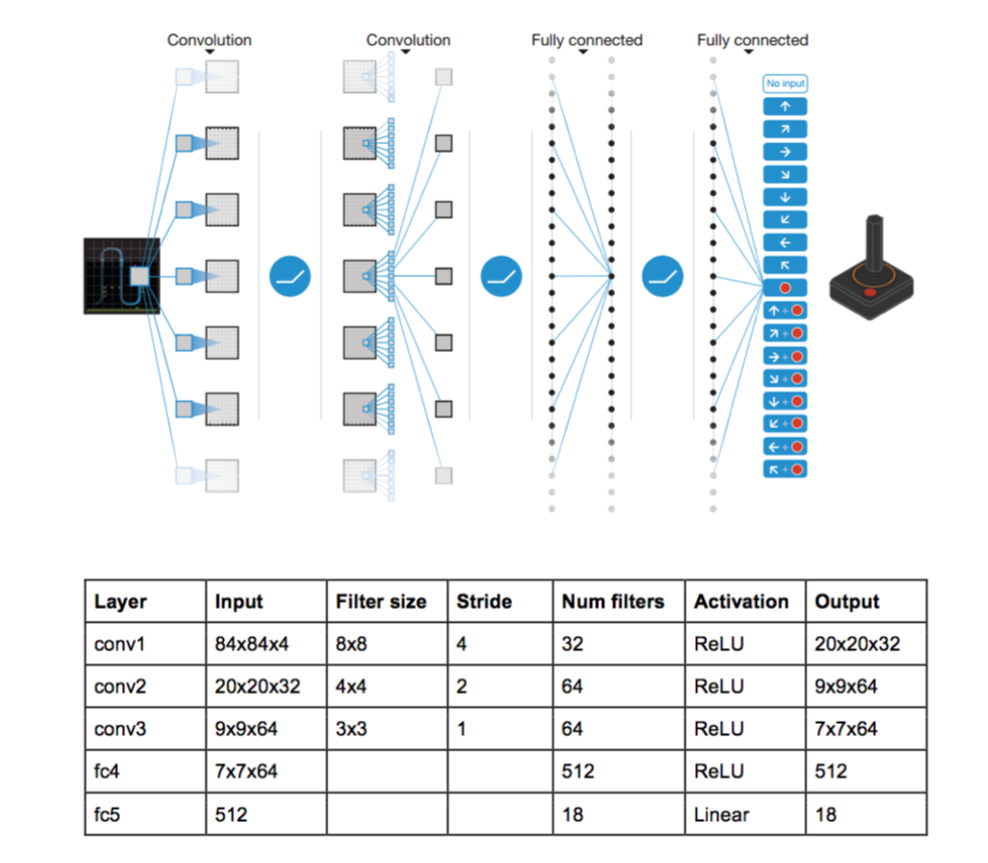Q 值可能是任意的实数，把对 Q 值的估计看作是机器学习中的回归问题，所以使用 平方误差损失(squared error loss) 作为优化的目标函数。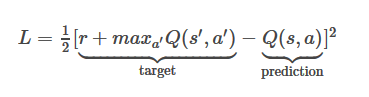$$L_{i}\left(\theta_{i}\right)=\mathbb{E}_{\left(s, a, r, s^{\prime}\right) \sim \mathrm{U}(D)}\left[\left(r+\gamma \max _{a^{\prime}} Q\left(s^{\prime}, a^{\prime} ; \theta_{i}^{-}\right)-Q\left(s, a ; \theta_{i}\right)\right)^{2}\right]$$

1. 输入当前状态 $s$，预测所有动作 $a$ 的 Q-值；
2. 输入下一状态 $s$，计算最大 $\max _{a^{\prime}} Q\left(s^{\prime}, a^{\prime}\right)$
3. 设置 Q 值优化目标为 $r+ \gamma \max _{a^{\prime}} Q\left(s^{\prime}, a^{\prime}\right)$
4. 反向传播更新网络 weights；

$$\nabla_{\theta_{i}} L\left(\theta_{i}\right)=\mathbb{E}_{s, a, r, s^{\prime}}\left[\left(r+\gamma \max _{a^{\prime}} Q\left(s^{\prime}, a^{\prime} ; \theta_{i}^{-}\right)-Q\left(s, a ; \theta_{i}\right)\right) \nabla_{\theta_{i}} Q\left(s, a ; \theta_{i}\right)\right]$$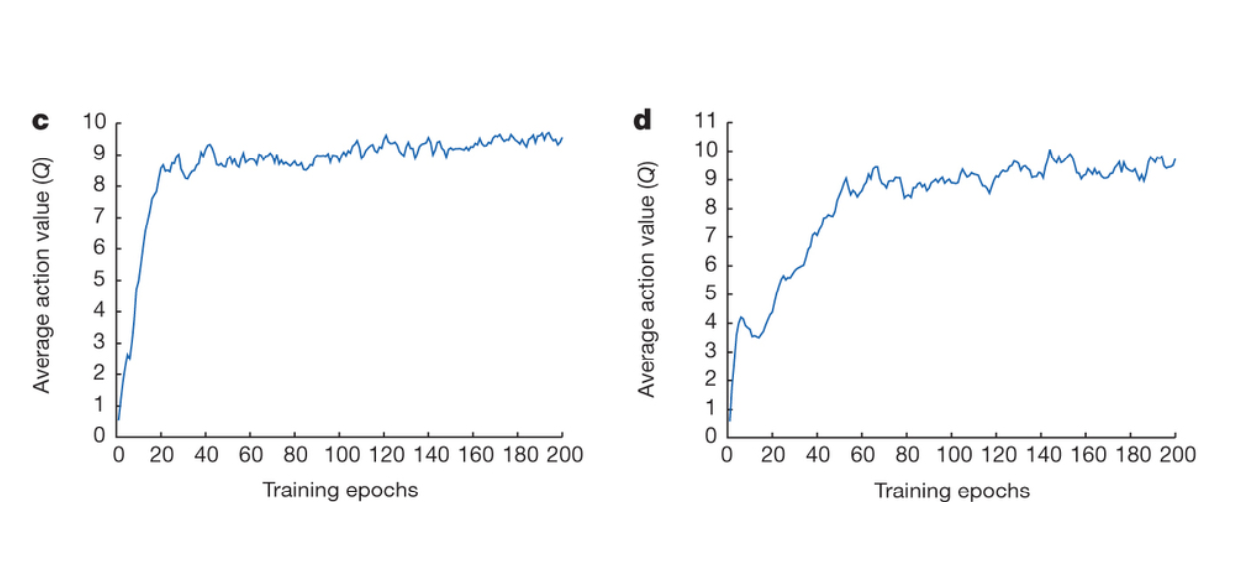• 网络是健忘的